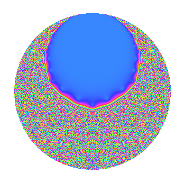# Properties

 Label 3.23.bLevel 3 Weight 23 Character orbit b Rep. character $$\chi_{3}(2,\cdot)$$ Character field $$\Q$$ Dimension 6 Newforms 1 Sturm bound 7 Trace bound 0

# Related objects

## Defining parameters

 Level: $$N$$ = $$3$$ Weight: $$k$$ = $$23$$ Character orbit: $$[\chi]$$ = 3.b (of order $$2$$ and degree $$1$$) Character conductor: $$\operatorname{cond}(\chi)$$ = $$3$$ Character field: $$\Q$$ Newforms: $$1$$ Sturm bound: $$7$$ Trace bound: $$0$$

## Dimensions

The following table gives the dimensions of various subspaces of $$M_{23}(3, [\chi])$$.

Total New Old
Modular forms 8 8 0
Cusp forms 6 6 0
Eisenstein series 2 2 0

## Trace form

 $$6q$$ $$\mathstrut +\mathstrut 86670q^{3}$$ $$\mathstrut -\mathstrut 11258688q^{4}$$ $$\mathstrut +\mathstrut 293575104q^{6}$$ $$\mathstrut -\mathstrut 3447063060q^{7}$$ $$\mathstrut +\mathstrut 57339715158q^{9}$$ $$\mathstrut +\mathstrut O(q^{10})$$ $$6q$$ $$\mathstrut +\mathstrut 86670q^{3}$$ $$\mathstrut -\mathstrut 11258688q^{4}$$ $$\mathstrut +\mathstrut 293575104q^{6}$$ $$\mathstrut -\mathstrut 3447063060q^{7}$$ $$\mathstrut +\mathstrut 57339715158q^{9}$$ $$\mathstrut -\mathstrut 186869600640q^{10}$$ $$\mathstrut -\mathstrut 1325972980800q^{12}$$ $$\mathstrut +\mathstrut 2025132496860q^{13}$$ $$\mathstrut +\mathstrut 2628031314240q^{15}$$ $$\mathstrut -\mathstrut 9703467227136q^{16}$$ $$\mathstrut +\mathstrut 42127150135680q^{18}$$ $$\mathstrut +\mathstrut 100485688668636q^{19}$$ $$\mathstrut -\mathstrut 789079193287812q^{21}$$ $$\mathstrut +\mathstrut 1045890315676800q^{22}$$ $$\mathstrut -\mathstrut 2472467233277952q^{24}$$ $$\mathstrut +\mathstrut 1396569263732790q^{25}$$ $$\mathstrut -\mathstrut 10731657558901410q^{27}$$ $$\mathstrut +\mathstrut 44570708098492800q^{28}$$ $$\mathstrut -\mathstrut 116169123436801920q^{30}$$ $$\mathstrut +\mathstrut 114109662401153292q^{31}$$ $$\mathstrut -\mathstrut 174850022773609920q^{33}$$ $$\mathstrut +\mathstrut 319017868488396288q^{34}$$ $$\mathstrut -\mathstrut 707589966262651200q^{36}$$ $$\mathstrut +\mathstrut 683782874080661820q^{37}$$ $$\mathstrut -\mathstrut 1038045209126993748q^{39}$$ $$\mathstrut +\mathstrut 1781949720499015680q^{40}$$ $$\mathstrut -\mathstrut 1108714403505421440q^{42}$$ $$\mathstrut +\mathstrut 273273330169769340q^{43}$$ $$\mathstrut +\mathstrut 3228622736179443840q^{45}$$ $$\mathstrut -\mathstrut 8349686917048426752q^{46}$$ $$\mathstrut +\mathstrut 10392767955626526720q^{48}$$ $$\mathstrut -\mathstrut 8294807529216677070q^{49}$$ $$\mathstrut +\mathstrut 14237699192275302144q^{51}$$ $$\mathstrut -\mathstrut 22636595958302682240q^{52}$$ $$\mathstrut +\mathstrut 48383805955937626944q^{54}$$ $$\mathstrut -\mathstrut 40874587160137441920q^{55}$$ $$\mathstrut +\mathstrut 43721391850797114540q^{57}$$ $$\mathstrut -\mathstrut 67285958146132360320q^{58}$$ $$\mathstrut -\mathstrut 44311576503733309440q^{60}$$ $$\mathstrut +\mathstrut 121649250388145492892q^{61}$$ $$\mathstrut -\mathstrut 184494903535705683060q^{63}$$ $$\mathstrut +\mathstrut 285203601894474055680q^{64}$$ $$\mathstrut -\mathstrut 374640791614422030720q^{66}$$ $$\mathstrut +\mathstrut 75701571086175260700q^{67}$$ $$\mathstrut -\mathstrut 233446021721582921856q^{69}$$ $$\mathstrut +\mathstrut 1113607014897000234240q^{70}$$ $$\mathstrut -\mathstrut 639860863731149721600q^{72}$$ $$\mathstrut -\mathstrut 57178481309533986900q^{73}$$ $$\mathstrut -\mathstrut 333973626119358339330q^{75}$$ $$\mathstrut -\mathstrut 731229802672243966080q^{76}$$ $$\mathstrut +\mathstrut 735750453969076417920q^{78}$$ $$\mathstrut +\mathstrut 384537395891327736396q^{79}$$ $$\mathstrut +\mathstrut 3109769787236091157926q^{81}$$ $$\mathstrut -\mathstrut 5166859472437001068800q^{82}$$ $$\mathstrut +\mathstrut 6698665889827068753792q^{84}$$ $$\mathstrut -\mathstrut 6772579853411844011520q^{85}$$ $$\mathstrut +\mathstrut 4995406256507248236480q^{87}$$ $$\mathstrut -\mathstrut 404168025320661381120q^{88}$$ $$\mathstrut -\mathstrut 3858783621100493362560q^{90}$$ $$\mathstrut -\mathstrut 2806742501496183911304q^{91}$$ $$\mathstrut +\mathstrut 2087220280517252053020q^{93}$$ $$\mathstrut +\mathstrut 8135725180445469439488q^{94}$$ $$\mathstrut -\mathstrut 15069021592890694238208q^{96}$$ $$\mathstrut +\mathstrut 1565231688506989945740q^{97}$$ $$\mathstrut -\mathstrut 6095367699696122981760q^{99}$$ $$\mathstrut +\mathstrut O(q^{100})$$

## Decomposition of $$S_{23}^{\mathrm{new}}(3, [\chi])$$ into irreducible Hecke orbits

Label Dim. $$A$$ Field CM Traces $q$-expansion
$$a_2$$ $$a_3$$ $$a_5$$ $$a_7$$
3.23.b.a $$6$$ $$9.201$$ $$\mathbb{Q}[x]/(x^{6} + \cdots)$$ None $$0$$ $$86670$$ $$0$$ $$-3447063060$$ $$q+\beta _{1}q^{2}+(14445-8\beta _{1}+\beta _{2})q^{3}+\cdots$$